## Turbulence

#### Video:

Not all flow is laminar.  In turbulent flow, water swirls erratically.  The velocity at a given point can change in magnitude and direction.  The onset of turbulent flow depends on the fluids speed, its viscosity, its density, and the size of the obstacle it encounters.  A single number, called the Reynolds number, can be used to predict the onset of turbulent flow.  For the flow past a smooth cylinder or sphere of diameter D or through a smooth tube with diameter D we have (approximately)

Reynolds number = (density * D * flow speed) / viscosity.

The Reynolds number has no units, the units on the right hand side of the equation all cancel out.  It increases with the flow speed and decreases with the viscosity.

Turbulence appears when the Reynolds number is about 2300.

#### Videos:

Under conditions of turbulent flow Bernoulli's equation is not applicable.  It was derived by equating the work done by pressure forces to the change in the potential energy and the ordered kinetic energy of the fluid.  Under conditions of turbulent flow the fluid gains disordered kinetic energy.  More work is done on the fluid and a greater pressure difference is needed to move the fluid at the same rate.Only recently have scientist been able to gain a better understanding of the patterns observed in turbulent flow under different circumstances.  The study of chaos is providing new insights into many related phenomena exhibiting turbulence, such as global weather patterns, the atmosphere of Jupiter, etc.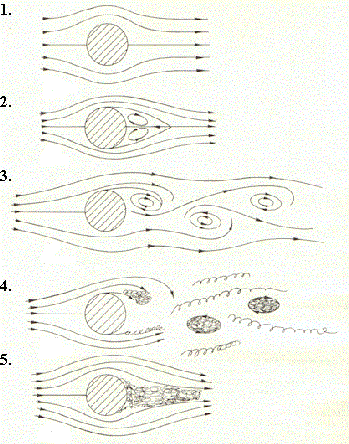Airflow past ordinary size objects is only laminar at low speed.  As the airspeed increases, a boundary layer forms.  This boundary layer fills the region behind the object with a turbulent wake.  The figure on the right shows the airflow past a cylinder as the airspeed and therefore the Reynolds number increase.

Remember:  Reynolds number = (density * D * flow speed) / viscosity.

In pictures 1-3 the Reynolds number is below 2000, in picture 4 it is approximately 10000, and in picture 5 it is above 100000.

• The first two pictures show laminar flow at low speed.  The air directly before and behind the cylinder comes to a stop.  The pressure is highest here, but the net force on the cylinder due to pressure differences is approximately zero.  There is no pressure drag due to pressure differences between the front and back side of the cylinder.  The cylinder experiences only viscous drag due to air friction.
• In the fourth picture a turbulent wake has formed.  The air behind the cylinder no longer slows down and the pressure no longer rises behind the cylinder.  Due to the high pressure in front of the cylinder it now experiences a large pressure drag.  This happens for Reynolds numbers of approximately 2000 to 100000.  The pressure drag is much larger than the viscous drag.  It can decrease the forward component of velocity of an object moving through a fluid or gas very rapidly.  A thrown object can seem to stop in midair and drop straight to the ground.  You can easily observe this by throwing an air-filled balloon.
• As the airspeed increases and the Reynolds number becomes larger than 100000, the turbulent region works itself forward as shown in the fifth picture.  We have what is called a turbulent boundary layer.  The flow lines now separate from the cylinder and follow the turbulent boundary layer, as shown in the fifth picture.  We have something similar to laminar flow around an object of a different shape.  The pressure behind the object rises again and the pressure drag is drastically reduced

#### Problem:

Calculate the Reynolds number for a ball with a 10 cm diameter thrown at 35 m/s through air with density of 1.25 kg/m3.
The viscosity of the air is 1.83*10-5 Pa-s.  Is the flow of the air around the ball laminar or turbulent?

Solution:

• Reasoning:
Turbulence appears when the Reynolds number is about 2300.
Reynolds number = (density * D * flow speed) / viscosity.
• Details of the calculation:
Reynolds number = (1.25 kg/m3)*(0.1 m)*(35 m/s)/(1.83*10-5 N s/m2) = 2.39*105.
The flow is turbulent.  The flow speed is high enough to imply a turbulent wake.

The condition of the surface determines when the Reynolds number becomes critical a turbulent boundary layer forms, and pressure drag is drastically reduced.  The rougher the surface, the lower the speed at which the Reynolds number becomes critical.  The surface of many balls used in a variety of sports is intentionally roughed up.  Golf balls have dimples and grooves, tennis balls have hair, etc.  This decreases the speed at which the Reynolds number becomes critical, and a turbulent boundary layer forms even at moderately high speeds.  In this way pressure drag can be largely eliminated and only the viscous drag acts on the ball.Reynolds number = 15000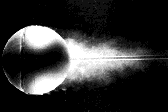Reynolds number = 30000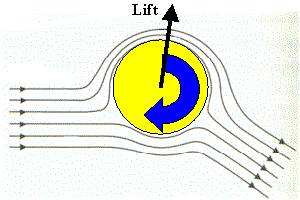The shape of an object can redirect airflow, thus producing lift.  Spinning symmetrical objects can also produce lift.  Even for laminar flow, a thin layer of air next to the object does not move with respect to the object.  A thin layer of air next to a spinning ball spins with the ball.  As the distance from the ball increases, the speed of the air changes, so that the airflow around the ball exhibits the patterns shown in the figure on the right.

If the ball is spinning clockwise as seen in the diagram, the air flows faster over the top of the ball.  The pressure on the top is lower than the pressure on the bottom of the ball, and there is a net lift force on the ball towards the top of the diagram.  This force is called the Magnus Force.  Note: The direction of the lift force depends on the direction of the ball's spin.  The lift force does not have to point upwards.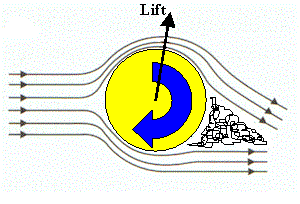Turbulent regions can also form.  The wake behind the ball can be deflected because the spinning surface pulls air with it.  This again can result in a net lift force.  This force is called the wake deflection force.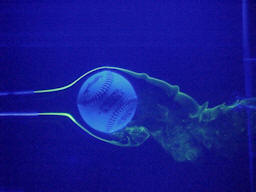Spinning baseball in a water tunnel

We can use Newton's third law to explain the lift force on the ball.
The spinning ball interacts with the air molecules.  The total momentum of interacting objects is conserved.  If the air molecules are deflected in one direction, then the ball must be deflected in the other direction.

#### Problem:

Suppose you are practicing baseball and throw the ball with a spin so that it moves forward and curves to the right.  Now when the actual game takes place you are pitching into the wind.  Please explain how you could alter your throw so as to make the ball take the same path.

Solution:

• Reasoning:
Drag resistance is responsible for slowing down the ball, and it depends on the ball's air speed.  Since there is a head wind the ball has to overcome the extra resistance, so you need to throw it harder than you did before.  But now the ball's speed in the air is faster so it needs to spin it less because the amount of deflection due to spinning depends on the ball's air speed as well as the spin rate, i.e. on the relative speed of the air with respect to the surface.

### Semi-laminar flow

#### Demonstration:

A ping-pong ball is suspended in the air flow from a hair dryer.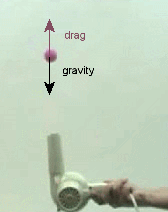When the air streams symmetrically around the ball in the upward direction, air drag and gravity point in opposite directions, and drag balances gravity.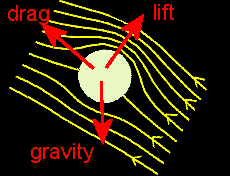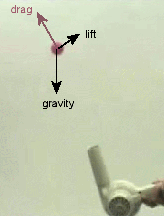When the air flow makes an angle with the vertical, drag and gravity do no longer point in exactly opposite directions, and drag alone cannot balance gravity.  The ball starts to drop out of the stream.  This redirects the stream and air has to flow faster over the upper surface of the ball, creating lift.  Lift and drag together now can balance gravity.

### Wings

An airplane's weight always acts in the downward direction.  By interacting with the surrounding air, the airplane's wings push some of the air moving relative to them downward.  The reaction force (Newton's third law) pushes the wings and therefore the airplane in the upward direction.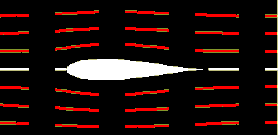The interaction between the air and a wing is complicated.  The air is a viscous fluid flowing with high speed around the wing.  The Reynolds number is certainly much larger than 100000 and a turbulent boundary later forms.  A wing shaped like an airfoil can prevent the turbulent boundary layer from separating over a large range of air speeds.  The remaining air then flows past this boundary layer in approximately laminar flow.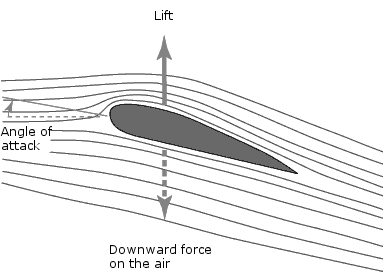To generate lift with a symmetric airfoil, the airfoil must be turned, so that its symmetry axis makes an angle with the direction of the airflow.  This angle is called the angle of attack of the wing with respect to the free-stream flow.

The airfoil now deflects the airflow downward and the reaction force pushes the airfoil upward.  The air also flows faster over the upper surface than over the lower surface.  The pressure therefore is lower near the top than near the bottom of the wing.  Again we conclude that the wing is pushed upward.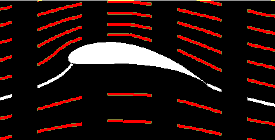Lift can also be generated by giving the airfoil an asymmetric shape.  Again , the airflow is deflected and the air flows faster over the upper surface than over the lower surface.  The wing is pushed up.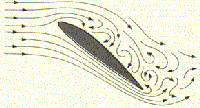The relative velocity of airplane and air is the most important factor in producing lift.  The lift will increase proportional to the square of the velocity.  Increasing the angle of attack increases the lift, but also increases the drag.  When the airspeed falls below a critical speed, (i.e. the Reynolds number falls below approximately 100000), a turbulent wake begins to detach.  The wing looses all lift, and pressure drag increases dramatically.  The airplane stalls, and without intervention by the pilot falls nearly straight to the ground.